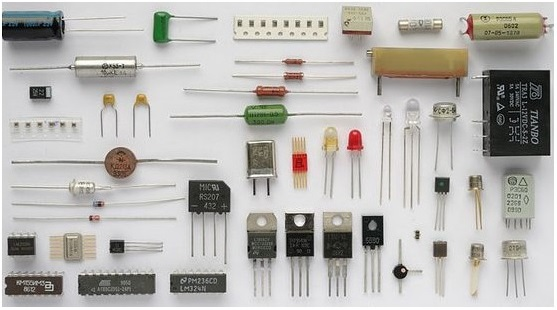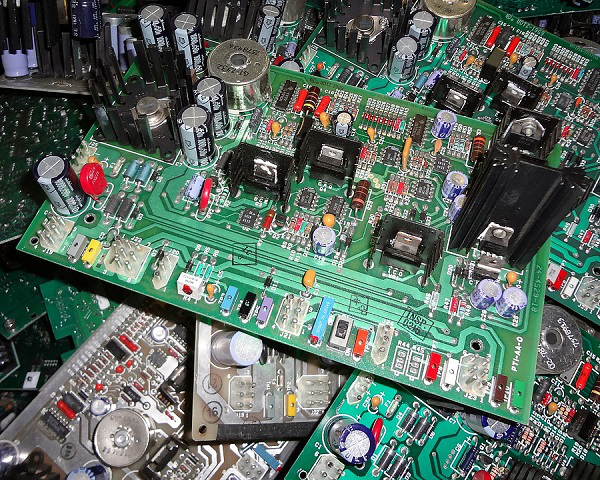# Electronic Circuits - Introduction

In Electronics, we have different components that serve different purposes. There are various elements which are used in many types of circuits depending on the applications.

## Electronic Components

Similar to a brick that constructs a wall, a component is the basic brick of a circuit. A Component is a basic element that contributes for the development of an idea into a circuit for execution.

Each component has a few basic properties and the component behaves accordingly. It depends on the motto of the developer to use them for the construction of the intended circuit. The following image shows a few examples of electronic components that are used in different electronic circuits.Just to gather an idea, let us look at the types of Components. They can either be Active Components or Passive Components.

### Active Components

• Active Components are those which conduct upon providing some external energy.

• Active Components produce energy in the form of voltage or current.

• Examples − Diodes, Transistors, Transformers, etc.

### Passive Components

• Passive components are those which start their operation once they are connected. No external energy is needed for their operation.

• Passive components store and maintain energy in the form of voltage or current.

• Examples − Resistors, Capacitors, Inductors, etc.

We also have another classification as Linear and Non-Linear elements.

### Linear Components

• Linear elements or components are the ones that have linear relationship between current and voltage.

• The parameters of linear elements are not changed with respect to current and voltage.

• Examples − Diodes, Transistors, Transformers, etc.

### Non-linear Components

• Non-linear elements or components are the ones that have a non-linear relationship between current and voltage.

• The parameters of non-linear elements are changed with respect to current and voltage.

• Examples − Resistors, Capacitors, Inductors, etc.

These are the components intended for various purposes, which altogether can perform a preferred task for which they are built. Such a combination of different components is known as a Circuit.

## Electronic Circuits

A certain number of components when connected on a purpose in a specific fashion makes a circuit. A circuit is a network of different components. There are different types of circuits.

The following image shows different types of electronic circuits. It shows Printed Circuit Boards which are a group of electronic circuits connected on a board.Electronic circuits can be grouped under different categories depending upon their operation, connection, structure, etc. Let’s discuss more about the types of Electronic Circuits.

### Active Circuit

• A circuit that is build using Active components is called as Active Circuit.

• It usually contains a power source from which the circuit extracts more power and delivers it to the load.

• Additional Power is added to the output and hence output power is always greater than the input power applied.

• The power gain will always be greater than unity.

### Passive Circuit

• A circuit that is build using Passive components is called as Passive Circuit.

• Even if it contains a power source, the circuit does not extract any power.

• Additional Power is not added to the output and hence output power is always less than the input power applied.

• The power gain will always be less than unity.

Electronic circuits can also be classified as Analog, Digital, or Mixed.

### Analog Circuit

• An analog circuit can be one which has linear components in it. Hence it is a linear circuit.

• An analog circuit has analog signal inputs which are continuous range of voltages.

### Digital Circuit

• A digital circuit can be one which has non-linear components in it. Hence it is a non-linear circuit.

• It can process digital signals only.

• A digital circuit has digital signal inputs which are discrete values.

### Mixed Signal Circuit

• A mixed signal circuit can be one which has both linear and non-linear components in it. Hence it is called as a mixed signal circuit.

• These circuits consist of analog circuitry along with microprocessors to process the input.

Depending upon the type of connection, circuits can be classified as either Series Circuit or Parallel Circuit. A Series Circuit is one which is connected in series and a parallel circuit is one which has its components connected in parallel.

Now that we have a basic idea about electronic components, let us move on and discuss their purpose which will help us build better circuits for different applications. Whatever might be the purpose of an electronic circuit (to process, to send, to receive, to analyze), the process is carried out in the form of signals. In the next chapter, we will discuss the signals and the type of signals present in electronic circuits.